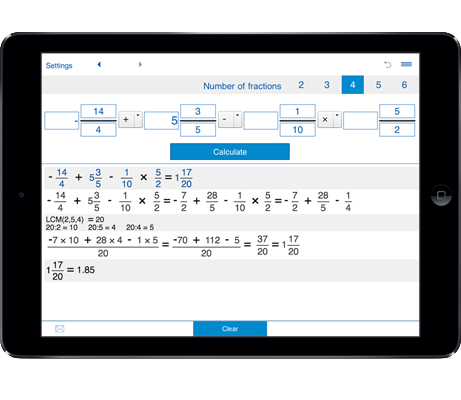# Subtracting fractions calculator

Fraction calculator for up to six fractions.
Basic calculations: addition, subtraction, multiplication and division.
Supports fractions, mixed numbers, and whole numbers.
History tape to view your recent calculations.

## Subtracting fractions calculator - subtract fractions, mixed fractions, and whole numbers

Subtracting fractions calculator lets you subtract fractions with different denominators, you can subtract fractions with like and unlike denominators, as well as subtract fractions with mixed numbers and whole numbers.
The subtracting fractions calculator allows you to subtract from two to six fractions.

### How to use the subtracting fractions calculator

1. Select the number of fractions for subtracting - 2 fractions, 3 fractions, 4 fractions, 5 fractions, or 6 fractions.

2. Enter the fractions, mixed fractions or whole numbers and click the Calculate button.